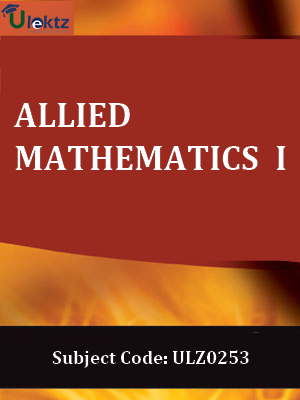•My WalletMy Order
•My Profile
•My Connections
•My Books
•My Videos
•My Tests
•My Calender
•My Messages
•My Shopping Cart
•My Orders
•Account Settings
•Help

# Book Details# Allied Mathematics – I

 Course Code : ULZ0253 Author : uLektz University : General for All University Regulation : 2017 Categories : Computer Science Format :ePUB3 (DRM Protected) Type : eBook

FREE

Description :Allied Mathematics – I of ULZ0253 covers the latest syllabus prescribed by General for All University for regulation 2017. Author: uLektz, Published by uLektz Learning Solutions Private Limited.

Note : No printed book. Only ebook. Access eBook using uLektz apps for Android, iOS and Windows Desktop PC.

##### Topics
###### UNIT I THEORY OF EQUATIONS

1.1 Theory of equations - Roots of an equation - Relation between roots and coefficients

1.2 Transformation of Equations - Increasing and decreasing the roots of an equation - Reciprocal equation

1.3 Horner’s method - Newtons method of finding roots

###### UNIT II MATRICES

2.1 Matrices: Characteristic roots and characteristic vectors - Properties (Statements only)

2.2 Cayley Hamilton theorem (Statement only) - Verification – To find the inverse using the above theorem - Diagonalization of a matrix and using it to find the powers of a matrix

###### UNIT III TRIGONOMETRY

3.1 Trigonometry – Expansions of Cos nθ, Sin nθ - Expressions of Cosθ, Sinθ, Tanθ in powers of θ

3.2 Hyperbolic functions - Inverse hyperbolic function - Real and imaginary parts of Sin (α + iβ), Cos (α + iβ), tan (α + iβ), tan-1 (α + iβ)

###### UNIT IV MULTIPLE INTEGRALS

4.1 Multiple integrals - Application of double integral - Integral in evaluating area between curves

4.2 Evaluation of triple integrals - Jacobian of two and three variables

4.3 Beta and Gamma functions – Relation - Evaluation of double and triple integrals using Beta and Gamma functions

###### UNIT V FOURIER SERIES

5.1 Fourier series – Definition - Finding Fourier coefficients for a given periodic function with period 2π

5.2 Odd and even function - Half range series